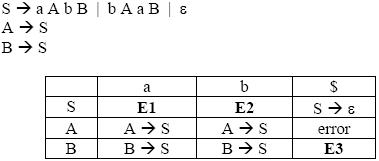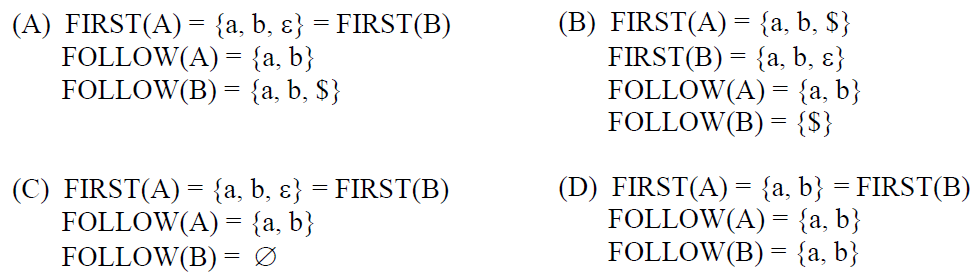Related Articles

# GATE | GATE CS 2012 | Question 65

• Difficulty Level : Basic
• Last Updated : 28 Jun, 2021

For the grammar below, a partial LL(1) parsing table is also presented along with the grammar. Entries that need to be filled are indicated as E1, E2, and E3.is the empty string, $indicates end of input, and, | separates alternate right hand sides of productions.(A) A (B) B (C) C (D) D Answer: (A) Explanation: First(X) - It is the set of terminals that begin the strings derivable from X. Follow(X) - It is the set of terminals that can appear immediately to the right of X in some sentential form. Now in the above question, FIRST(S) = { a, b, epsilon} FIRST(A) = FIRST(S) = { a, b, epsilon} FIRST(B) = FIRST(S) = { a, b, epsilon} FOLLOW (A) = { b , a } FOLLOW (S) = {$ } U FOLLOW (A) = { b , a , $} FOLLOW (B) = FOLLOW (S) = { b ,a ,$ }

epsilon corresponds to empty string.

Attention reader! Don’t stop learning now.  Practice GATE exam well before the actual exam with the subject-wise and overall quizzes available in GATE Test Series Course.

Learn all GATE CS concepts with Free Live Classes on our youtube channel.

My Personal Notes arrow_drop_up